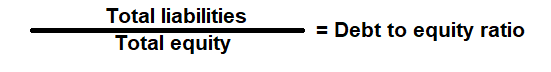# Debt Equity Ratio Calculator

Key in the Total Liabilities and Total Equity Values from your Balance Sheet to the respective fields given below and then click Calculate to get the desired result.

# Debt to Equity Ratio Calculator - Glossary:

Debt to Equity Ratio: Shows the proportion of Total Liabilities to Total Equity. In simple words, it measures the riskiness of a company's financial structure.

### Formula:### How to use this equation?

This is a balance sheet component; the values are commonly stated against Total Liabilities and Total Equity. To use this ratio, divide the total liabilities by total equity.

Total Liabilities (Debt):
Total Liabilities is the source of the funds such as short term and long-term external borrowing that is mainly used to fund its asset purchase.

Total Shareholder's Equity (Equity):
Total equity is the is the value left in the company after subtracting total liabilities from total assets.

Example:
Debt to equity (D/E) ratio for a company with a total liability of \$360,000 and total equity of \$720,000 is 0.5:1. It means that, the assets of the company are funded 2-to-1 by investors to creditors. In other words, the company has a \$0.50 of debt for every dollar of equity.